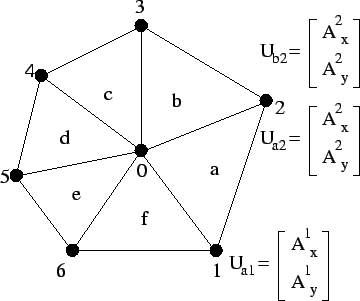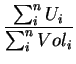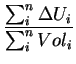Next: 4.3.1.7 Miscellaneous Features Up: 4.3.1 The Model Definition Previous: 4.3.1.5 Parameters

### 4.3.1.6 Auxiliaries

A very useful feature of AMIGOS is the definition of auxiliary variables. These variables are treated like quantities but are solved explicitly which reduces the amount of memory and calculation time. Explicit means, that they are not involved in the Newton iteration step solving the global stiffness matrix but they build a subsystem of their own which is defined by the interconnections of the nearest element neighbors of the mesh (Fig. 4.3.1.6).

AMIGOS automatically detects the dimension of the auxiliary variable that might be an n-dimensional tensor, and writes its output as separate result value to a file for later reuse or visualization.

Figure 4.5: Auxiliary calculation depending on element interconnections and weighting functionAux U = grad(A); # defines U as auxiliary # to calculate a gradient # field of quantity A Aux Vol = V/3; # defines Vol as auxiliary # for later use as weighting # function 

To meet all requirements of different auxiliary types there are several update modes that have already been introduced in the section about the Input & Control Interface (4.2). The following list shows the different modes and their calculation methods referring to Fig. 4.3.1.6 where U is a derived gradient field of the quantity A weighted by the volume of the surrounding elements corresponding to point 0 (e.g. volume might be a Voronoi box):

• if the user does not specify the type of update mode in the Input & Control Interface the default formula
 Aux U =(4.4)

will be used to calculate the auxiliaries.
• update Newton-like initializes the auxiliary U to be updated in the same manner as a quantity calculated by a Newton iteration where the used formula is
 Aux U =(4.5)

• update after timestep initializes the auxiliary U to be updated after finishing a time step integration. This means, that during the time-step calculation the auxiliary U is treated as a constant value. The used formula is the same as in (4.4).

• update after timestep but Newton-like handles the auxiliary U as described in the previous point but using the calculation after (4.5).

• explicit moving grid integration offers the possibility to write a calculated auxiliary variable to the coordinate vectors. In this case the auxiliary must be a vector of the size of element points and after each time-step the coordinates of each element are updated to the new value calculated during solving the total system.Next: 4.3.1.7 Miscellaneous Features Up: 4.3.1 The Model Definition Previous: 4.3.1.5 Parameters